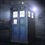# RMO practice 2

Prove that,$\dfrac{2}{a+b}+\dfrac{2}{b+c}+\dfrac{2}{c+a} \geq \dfrac{9}{a+b+c}\\a,b,c \in R^+$Note by Adarsh Kumar
5 years, 7 months ago

This discussion board is a place to discuss our Daily Challenges and the math and science related to those challenges. Explanations are more than just a solution — they should explain the steps and thinking strategies that you used to obtain the solution. Comments should further the discussion of math and science.

When posting on Brilliant:

• Use the emojis to react to an explanation, whether you're congratulating a job well done , or just really confused .
• Ask specific questions about the challenge or the steps in somebody's explanation. Well-posed questions can add a lot to the discussion, but posting "I don't understand!" doesn't help anyone.
• Try to contribute something new to the discussion, whether it is an extension, generalization or other idea related to the challenge.
• Stay on topic — we're all here to learn more about math and science, not to hear about your favorite get-rich-quick scheme or current world events.

MarkdownAppears as
*italics* or _italics_ italics
**bold** or __bold__ bold
- bulleted- list
• bulleted
• list
1. numbered2. list
1. numbered
2. list
Note: you must add a full line of space before and after lists for them to show up correctly
paragraph 1paragraph 2

paragraph 1

paragraph 2

[example link](https://brilliant.org)example link
> This is a quote
This is a quote
    # I indented these lines
# 4 spaces, and now they show
# up as a code block.

print "hello world"
# I indented these lines
# 4 spaces, and now they show
# up as a code block.

print "hello world"
MathAppears as
Remember to wrap math in $$ ... $$ or $ ... $ to ensure proper formatting.
2 \times 3 $2 \times 3$
2^{34} $2^{34}$
a_{i-1} $a_{i-1}$
\frac{2}{3} $\frac{2}{3}$
\sqrt{2} $\sqrt{2}$
\sum_{i=1}^3 $\sum_{i=1}^3$
\sin \theta $\sin \theta$
\boxed{123} $\boxed{123}$

## Comments

Sort by:

Top Newest

Using T2's Lemma,

$\dfrac{2}{a+b}+\dfrac{2}{b+c}+\dfrac{2}{c+a} = \dfrac{\left(\sqrt{2}\right)^2}{a+b}+\dfrac{\left(\sqrt{2}\right)^2}{b+c}+\dfrac{\left(\sqrt{2}\right)^2}{c+a} \geq \dfrac{\left(3\sqrt{2}\right)^2}{2(a+b+c)} = \dfrac{18}{2(a+b+c)}= \dfrac{9}{a+b+c} \ \Huge{\ddot\smile}$

- 5 years, 7 months ago

Log in to reply

HAAHAHHA T2!

- 5 years, 7 months ago

Log in to reply

Same method.

- 5 years, 7 months ago

Log in to reply

Nice!

- 5 years, 7 months ago

Log in to reply

I have a same way.

- 5 years, 7 months ago

Log in to reply

Do AM-HM on the numbers $a+b,b+c,c+a$.

- 5 years, 7 months ago

Log in to reply

T2 is a cuter method though :P

- 5 years, 7 months ago

Log in to reply

From your inequality, we have: $2(a+b+c)(\frac { 1 }{ a+b } +\frac { 1 }{ b+c } +\frac { 1 }{ c+a } )\ge 9$

1, We can use AM-GM for 3 numbers in left-hand side . 2, We can extract in left-hand side and use AM-GM for 2 numbers too ^_^

- 5 years, 7 months ago

Log in to reply

can someone tell me what is T2's Lemma?

- 5 years, 7 months ago

Log in to reply

- 5 years, 7 months ago

Log in to reply

Thank you ... how do u do by AM-HM inequality?

- 5 years, 7 months ago

Log in to reply

Power Mean (QAGH)

- 5 years, 7 months ago

Log in to reply

×

Problem Loading...

Note Loading...

Set Loading...